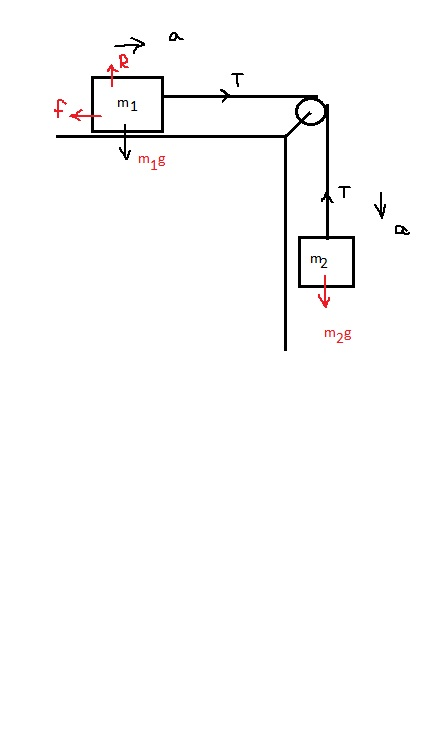# Objects with masses m1 = 11.0 kg and m2 = 7.0 kg are connected by a light string that passes over...

## Question:

Objects with masses m1 = 11.0 kg and m2 = 7.0 kg are connected by a light string that passes over a friction-less pulley as in the figure below.

If, when the system starts from rest, m2 falls 1.00 m in 1.49 s, determine the coefficient of kinetic friction between m1 and the table.

## Newton's Second law and Friction

According to Newton's second law, the net force on an object is given by the product of mass and acceleration of the object. If the object is at rest then force on it would be zero. And if the object is not in motion then static friction will come into act otherwise kinetic friction will be taking place.Given

Distance fall by mass 2 (S) = 1 m

time taken (t) = 1.49 s

Now using the kinematic equation

{eq}\displaystyle S = ut + 0.5at^{2}\\ 1 = 0 + 0.5*a*1.49^{2} \\ a = 0.9 \ m/s^{2} {/eq}

Now considering the FBD of mass 2

{eq}\displaystyle m_{2}g - T = m_{2}a \\ T = m_{2}(g-a) \\ T = 7 (9.81-0.9) = 62.364 \ N {/eq}

Now considering the FBD of mass 1

{eq}\displaystyle T - f = m_{1}a \\ 62.364 - (\mu_{k}m_{1}g) = m_{1}a \\ 62.364 - (\mu_{k}*11*9.81) = 11*0.9 \\ \mu_{k} = 0.486 {/eq}